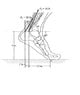# Statics 221. Determine moment.

• celtics777

## Homework Statement

Thee foot segment is subjected to the pull of the two plantarflexor muscles. Determine the moment of each force about the point of contact A on the ground.

## Homework Equations

M=Fd
I've used others to help determine certain angles, but I'm not sure if it was even necessary.

## The Attempt at a Solution

I have been able to calculate most of the angles, but I'm not exactly sure where to go from there. Which force do I use when?

Is it something like (20cos(60))(30cos(70))-(20sin(60))(30sin(70))? No idea if I'm on the right track or anything.

#### Attachments

•Statics3.jpg
13.1 KB · Views: 894
It is often best to break up the forces ino their x and y components, then determine the moment of each component about A by using the perpendicular distance from the line of action of that component force to A, and adding them up, being sure to note signage (cw or ccw). For example, looking at the 20 N force, its x comp is 20cos60 and its y component is 20sin60. The moment from the x component is 20cos60(4).. Now find the moment from the y component of that force, and add the moments together algebraically to get the moment of that 20 N force about A. Do the same for the other force.

Edit: I'm looking at the figure bit more closely, and it is wrong. The 60 degree angle is not compatible with the dimensions shown. Toss this problem out. But remember the 'force times perpendicular distance' concept for determining moments. I'm not sure about a bad prof, but I see what you mean about a bad book (could just be a fluke, though).

Last edited:
celtics777: The 60 deg angle label, and the diagonal line drawn from point A to F2, are irrelevant. Just ignore them, and solve the remaining problem. Study how to compute a moment.

An aternate mthod is to use the cross product rule for determining moments, M = r X F = (r)(F)(sin theta), where, when calculating the moment about A due to F2, r is the length of the position vector (the diagonal line shown), r = sq rt (3.5^2 + 4^2), F2 is given as 30, and theta is the interior angle between F2 and r, which is incorrectly shown as 130 degrees. If you do it that way, youll get the wrong answer because of the book error in labeling the figure. Or maybe the 60 degrees was intended to be correct, and the dimensions 3.5 and 4 are incorrect. Bad problem...it leads one to spin his or her heels in determining a solution to match the book answer which who knows is correct. Rip out the page, but remember the concept in determining moments.